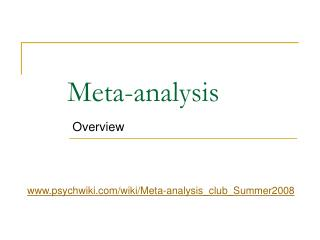DownloadDownload PresentationMeta-analysis

# Meta-analysis

Download Presentation## Meta-analysis

- - - - - - - - - - - - - - - - - - - - - - - - - - - E N D - - - - - - - - - - - - - - - - - - - - - - - - - - -
##### Presentation Transcript

1. Meta-analysis Overview www.psychwiki.com/wiki/Meta-analysis_club_Summer2008

2. Definition • Definition • A meta-analysis statistically combines the results of several studies that address a shared research hypotheses. • A study collects data from individual subjects (such as 100 subjects = 100 “data points”) • A meta-analysis collects data from individual studies (such as 100 studies = 100 “data points”)

3. Steps 1. Defines your hypothesis 2. Locate Studies 3. Find “effect size” for each study 4. Average the “effect sizes” together 5. If you want, you can analyze “moderators” 6. Write the manuscript

4. Why do a meta-analysis? • Easy • Steps are simple, there is software to calculate everything • Cost-effective • Since you have already read a bunch of articles to write a paper, not much more work to synthesize them together • Best type of article • Most highly cited type of article. • Advantages of both qualitative and quantitative research • Truly answers research questions within the literature (compared to single studies which can’t truly generalize)

5. Step 2 (again) • Locate studies • Techniques - database searches, ancestry approach, descendancy approach, hand searching, invisible college • Doesn’t have to be comprehensive (fail-safe n) but needs to be close to comprehensive • (create excel file – example) * so that you can Search it, copy/paste into References

6. Step 3 (again) • Find “effect size” for each study • (1) Decide: convert into “r” or “d” • (2) Download “es_calculator.zip” from http://mason.gmu.edu/~dwilsonb/ma.html • (3) Use “es_calculator” to calculate ES • (4) Record in excel file, separate row for each study • (excel file – example) * need to input sample size and ES

7. Step 4 (again) • Average the “effect sizes” together • Conceptually… • First, weight them by sample size/inverse variance • Second, sum them together • Third, divide by sum of total sample size. • In Practice… • You calculate inverse variance • Then use Macro to do the rest Download macro for SPSS http://mason.gmu.edu/~dwilsonb/ma.html

8. Step 5 (again) • If you want, you can analyze “moderators” • Conceptually… • Can test categorical moderators (categories like college student versus actual juror) similar to ANOVA • Can test continuous moderators (such as length of stimulus) similar to Regression • In Practice… • Use macros downloaded from http://mason.gmu.edu/~dwilsonb/ma.html • Macros exist for ANOVA & Regression

9. Some cool things about meta-analyses…